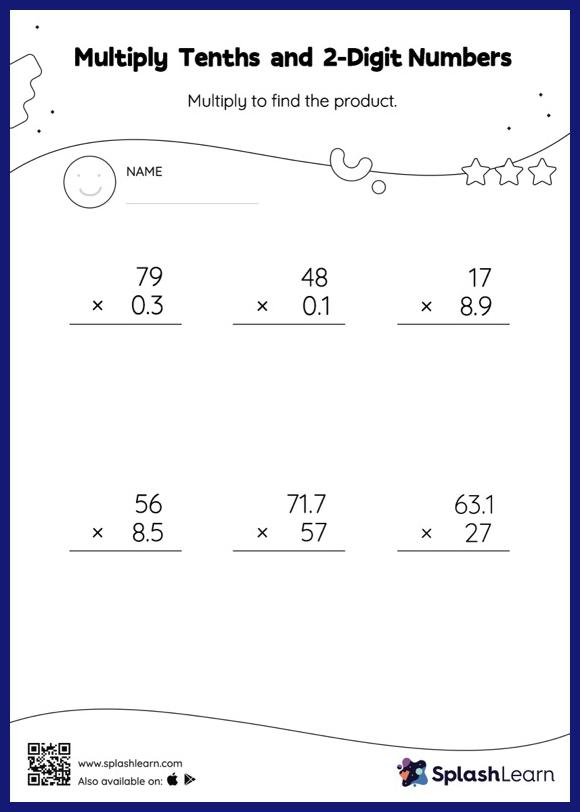# Multiply Tenths and 2-Digit Numbers: Vertical Multiplication Worksheet

Home > Multiply Tenths and 2-Digit Numbers: Vertical MultiplicationLearners hone their math skills with this multiply tenths and 2-digit numbers worksheet. When multiplying a decimal by a two-digit number, students may ignore the decimal point during calculations and bring it back when writing the result. This multiply tenths and 2-digit numbers worksheet provides plenty of practice with this concept.